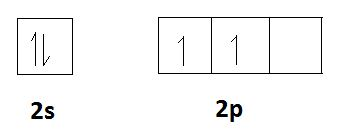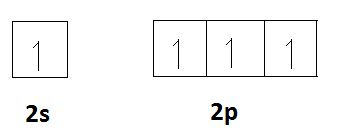# Difference Between Valency and Covalency

## Main Difference – Valency vs Covalency

An atom is the building block of matter. Each and every atom is composed of a nucleus and an electron cloud. The nucleus is the core of the atom and it is surrounded by the electron cloud. The concept of electron cloud is evolved based on the probability of the position of an electron. This means an electron is always in motion around the nucleus. This pathway is called an orbital or shell. The electrons are said to be moving along these orbitals. The valency and covalency are two terms that are related to the number of electrons present in an atom. The main difference between valency and covalency is that valency is the number of electrons that an atom will lose or gain in order to stabilize itself whereas covalency is the maximum number of covalent bonds that an atom can form using its empty orbitals.

### Key Areas Covered

1. What is Valency
– Definition, Properties, Examples
2. What is Covalency
– Definition, Properties, Examples
3. What is the Difference Between Valency and Covalency
– Comparison of Key Differences

Key Terms: Atom, Covalency, Covalent Bond, Electron, Orbital, Shell, Valency## What is Valency

Valency can be defined as the number of electrons that an atom will lose or gain in order to stabilize itself. The electrons in the outermost orbital of an atom are known as valence electrons. Sometimes, the number of valence electrons is considered as the valency of that element. For example, the valency of Hydrogen (H) is 1 since the hydrogen atom can be stabilized either by losing or gaining 1 electron. Chlorine atom has 7 electrons in the outermost orbital (number of valence electrons is 7) but by gaining 1 more electron, it can obtain the noble gas electron configuration of Argon (Ar) which is more stable. It is easy to obtain one electron rather than losing 7 electrons, so the valency of Chlorine is considered as 1.

The electron configuration of an element gives the valency of a particular element. The following table shows some of the elements with their valancies.

 Element Electron configuration Electrons required to be obtained or released to obey octet rule Valency Sodium (Na) 1s22s22p63s1 (-)1 1 Calcium (Ca) 1s22s22p63s23p64s2 (-)2 2 Nitrogen (N) 1s22s22p3 (+)3 3 Chlorine (Cl) 1s22s22p63s23p5 (+)1 1

The above table shows the valency of some elements. There, the (-) mark indicated the number of electrons that have to be removed in order to get stabilized. The (+) mark indicates the number of electrons that have to be obtained in order to get stabilized.Figure 1: The periodic table of elements

Apart from that, the periodic table of elements also can give an idea about the valency of an element. The group 1 elements always have valency 1 and for group 2 elements, the valency is 2.

## What is Covalency

Covalency is the maximum number of covalent bonds that an atom can form using its empty orbitals. Covalency depends on the number of valence electrons of an element. For example, the number of valence electrons present in Hydrogen is 1 and the covalency of Hydrogen is also 1 since it has only one electron that can be shared with another atom to form a covalent bond.

If an element such as carbon is considered, the electron configuration of carbon is 1s22s22p2. The number of valence electrons of carbon is 4. It has empty p orbitals. Therefore, the two s electrons in 2s orbital can be separated and included in these p orbitals. Then there are 4 unpaired electrons in carbon. Thus, carbon has 4 electrons to be shared in order to form covalent bonds. Therefore, the covalency of carbon is 4. It is the maximum number of covalent bonds that a carbon atom can have. This is explained by orbital diagrams shown below.

The valence electrons of carbon;The spread of electrons to the empty orbitals;Now there are 4 unpaired electrons for carbon to share with other atoms in order to form covalent bonds.

## Difference Between Valency and Covalency

### Definition

Valency: Valency is the number of electrons that an atom will lose or gain in order to stabilize itself.

Covalency: Covalency is the maximum number of covalent bonds that an atom can form using its empty orbitals.

### Relationship with Valence Electrons

Valency: Valency can be either equal to the number of valence electrons or not.

Covalency: Covalency depends on the number of valence electrons.

### Empty Orbitals

Valency: Valency gives the number of electrons required to fill the empty orbitals.

Covalency: Covalency depends on the number of empty orbitals present in an atom.

### Type of Bonding

Valency: Valency can be given for elements that can form either ionic or covalent bonds.

Covalency: Covalency can be given only for elements that can form covalent bonds.

### Conclusion

The valency may sometimes equal the number of valence electrons of an atom, but most often they are different. However, covalency is totally dependent on the number of valence electrons of an atom. That is because valence electrons determine the number of covalent bonds that an atom can have. Thus, it is important to know the difference between valency and covalency.

##### References:

1. “Covalency.” Chemistry-covalency and molecular structures. N.p., n.d. Web. Available here. 18 July 2017.
2. “Valence (chemistry).” Wikipedia. Wikimedia Foundation, 08 July 2017. Web. Available here. 18 July 2017.

##### Image Courtesy:

1. “Periodic-table” By LeVanHan – Own work (CC BY-SA 3.0) via Commons Wikimedia